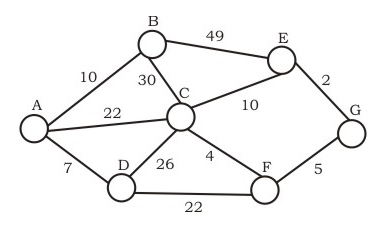# GATE | GATE-IT-2004 | Question 57

Consider the undirected graph below:Using Prim’s algorithm to construct a minimum spanning tree starting with node A, which one of the following sequences of edges represents a possible order in which the edges would be added to construct the minimum spanning tree?
(A) (E, G), (C, F), (F, G), (A, D), (A, B), (A, C)
(B) (A, D), (A, B), (A, C), (C, F), (G, E), (F, G)
(C) (A, B), (A, D), (D, F), (F, G), (G, E), (F, C)
(D) (A, D), (A, B), (D, F), (F, C), (F, G), (G, E)

Explanation: A and B are False  : The idea behind Prim’s algorithm is to construct a spanning tree – means all vertices must be connected but here vertices are disconnected

C. False. Prim’s is a greedy algorithm and At every step, it considers all the edges that connect the two sets, and picks the minimum weight edge from these edges. In this option weight of AB<AD so must be picked up first.

D.TRUE. It represents a possible order in which the edges would be added to construct the minimum spanning tree. Prim’s algorithm is also a Greedy algorithm. It starts with an empty spanning tree. The idea is to maintain two sets of vertices. The first set contains the vertices already included in the MST, the other set contains the vertices not yet included. At every step, it considers all the edges that connect the two sets, and picks the minimum weight edge from these edges. After picking the edge, it moves the other endpoint of the edge to the set containing MST. Read more at: https://www.geeksforgeeks.org/greedy-algorithms-set-5-prims-minimum-spanning-tree-mst-2/

My Personal Notes arrow_drop_up
Article Tags :

Be the First to upvote.

Please write to us at contribute@geeksforgeeks.org to report any issue with the above content.